﻿ 船用空调器振动及隔振措施分析
 舰船科学技术2020, Vol. 42Issue (6): 71-74    DOI: 10.3404/j.issn.1672-7649.2020.06.014PDF

1. 海军驻大连地区第二军事代表室，辽宁 大连 116005;
2. 中国舰船研究设计中心船舶振动噪声重点实验室，湖北 武汉 430064

The analysis of vibration and isolation measures of air conditioner on ship
MO Li-xin1, CHEN Pan2, ZHOU Jian2
1. No.2 Military Representative Office of the Navy in Dalian Area Dalian 116005, China;
2. China Ship Development and Design Center, National Key Laboratory on Ship Vibration and Noise, Wuhan 430064, China
Abstract: Air conditioner is one of equipments widely used on ship. Its vibration state not only affects the living environment of the surrounding accommodation rooms, but also affects the vibration state of ship. This paper carried out vibration test and analysis on an air conditioner, and on the basis of actual running state, the vibration isolation performance of single-layer vibration isolation of fan, single-layer vibration isolation of air conditioner and double-layer vibration isolation of air conditioner were analyzed, and the influence of three conditions on air conditioner vibration transmission were analyzed as well. According to comparing and analyzing, the three methods can effectively reduce the air conditioner vibration transfer to the install foundation, the vibration isolation performance of double-layer vibration isolation is the best, and can effectively reduce the low frequency vibration transmission.
Key words: Air conditioner     vibration     single layer vibration isolation     double-layer vibration isolation
0 引言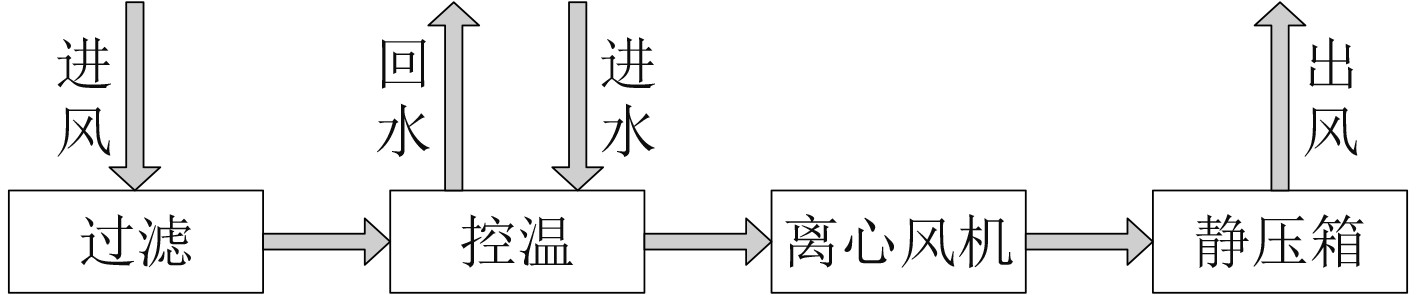图 1 空调器空气流通图 Fig. 1 Diagram of air flow with air conditioner

1 典型空调器减振措施分析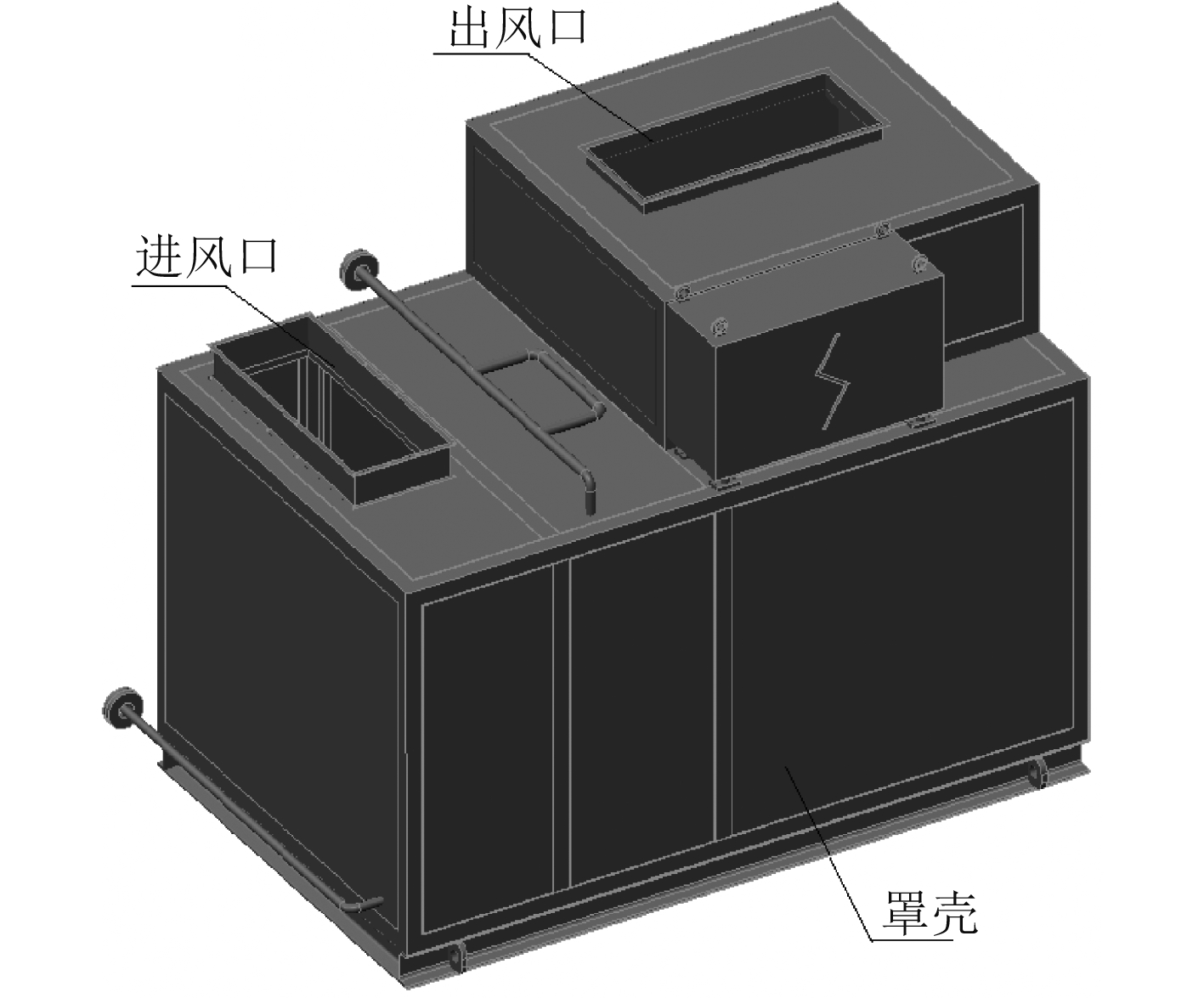图 2 典型空调器三维图 Fig. 2 Three-dimensional diagram of typical air conditioner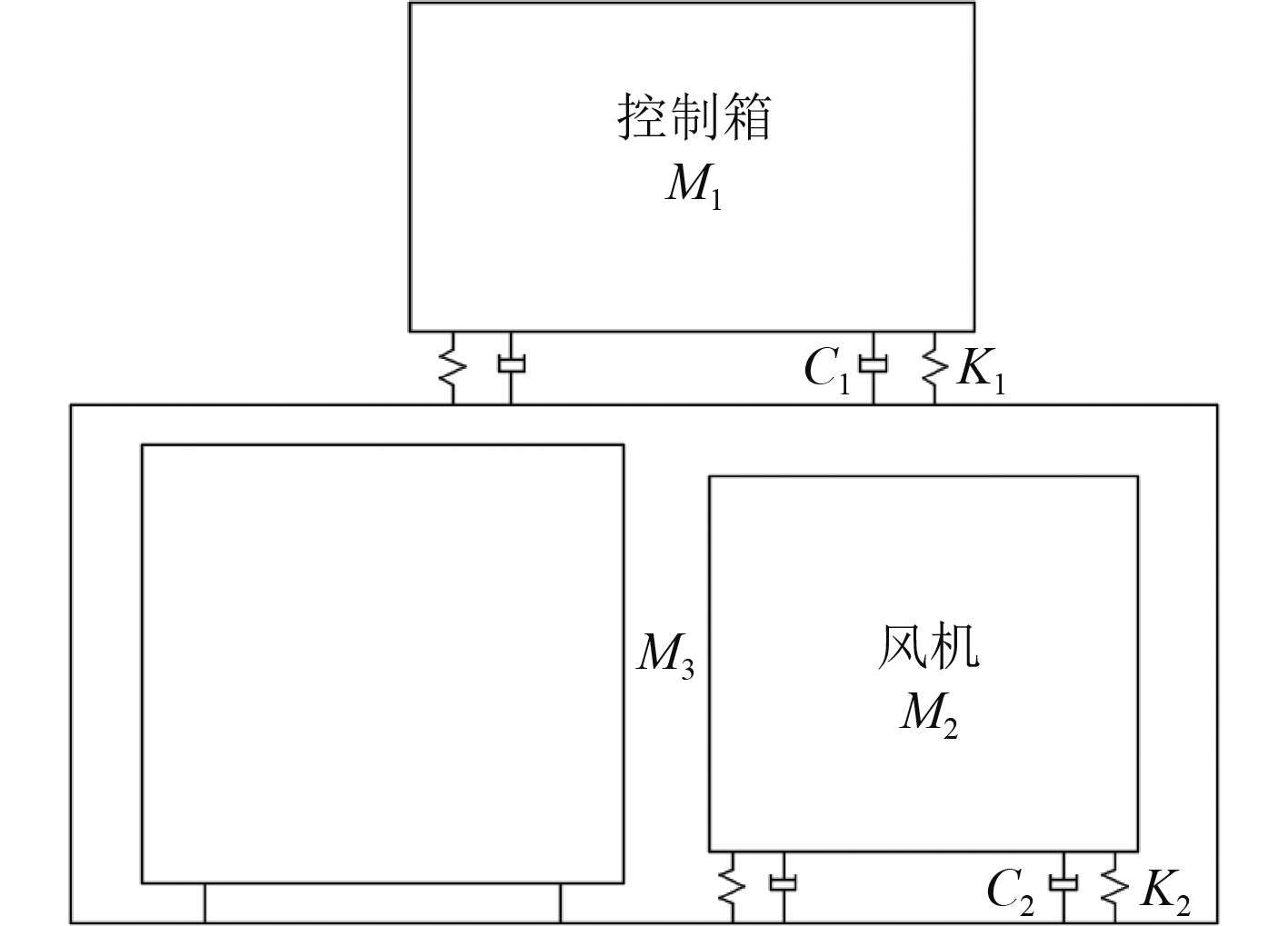图 3 空调器风机隔振模型 Fig. 3 Model of signal layer vibration isolation of fan

 $m\ddot x + C\dot x + Kx = F\left( t \right) = {F_0}\sin \omega t\text{，}$ (1)

 ${T_A} = \sqrt {\frac{{1 + {{\left[ {2 \cdot \zeta \cdot \left( {\displaystyle\frac{\omega }{{{\omega _n}}}} \right)} \right]}^{\rm{2}}}}}{{{{\left[ {1 - {{\left( {\displaystyle\frac{\omega }{{{\omega _n}}}} \right)}^2}} \right]}^{\rm{2}}} + {{\left[ {2 \cdot \zeta \cdot \left( {\displaystyle\frac{\omega }{{{\omega _n}}}} \right)} \right]}^{\rm{2}}}}}} \text{。}$ (2)

2 典型空调器振动测试分析及分析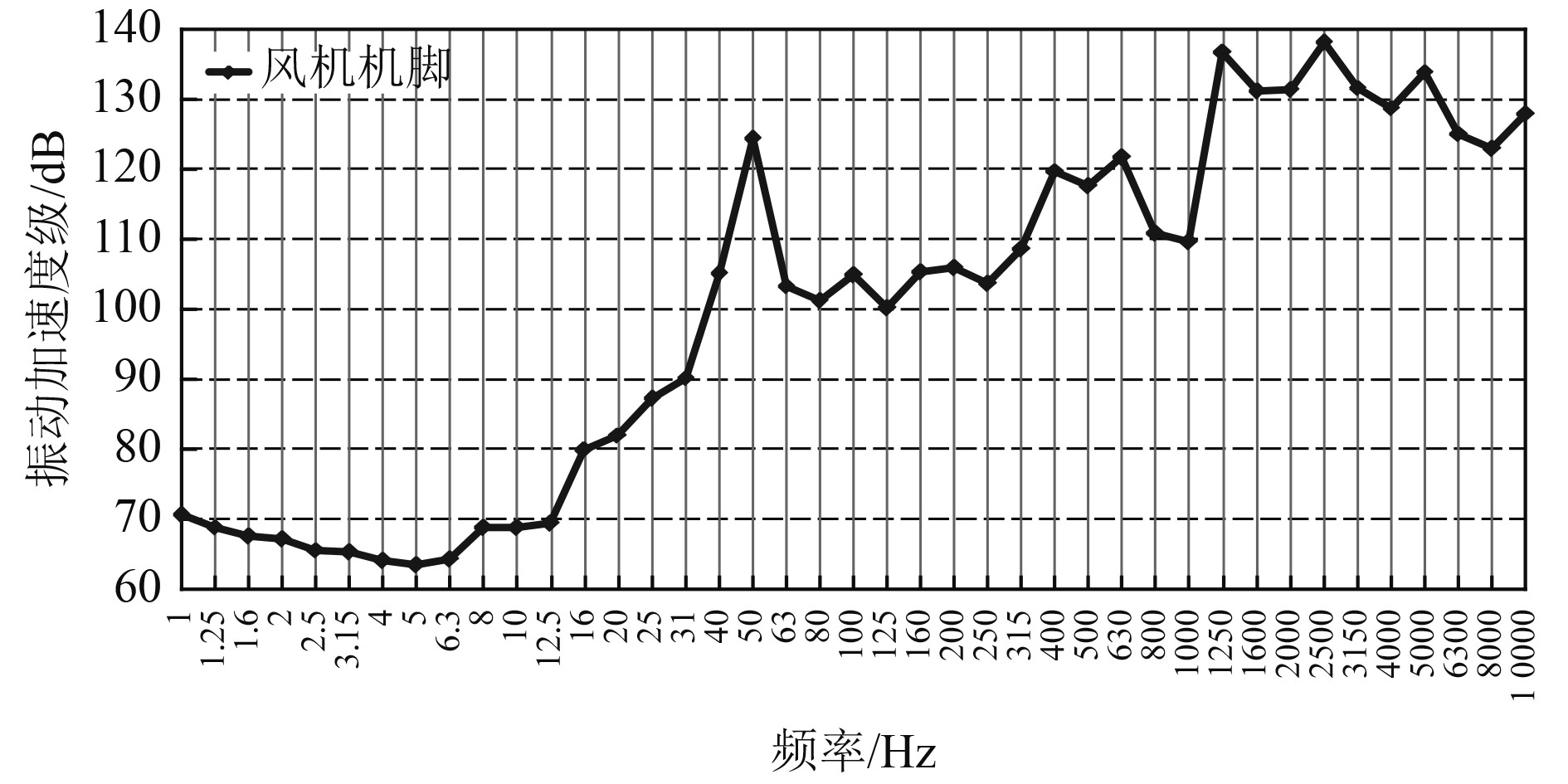图 4 离心风机机脚振动加速度级曲线 Fig. 4 Curve of vibration acceleration level of feet in fan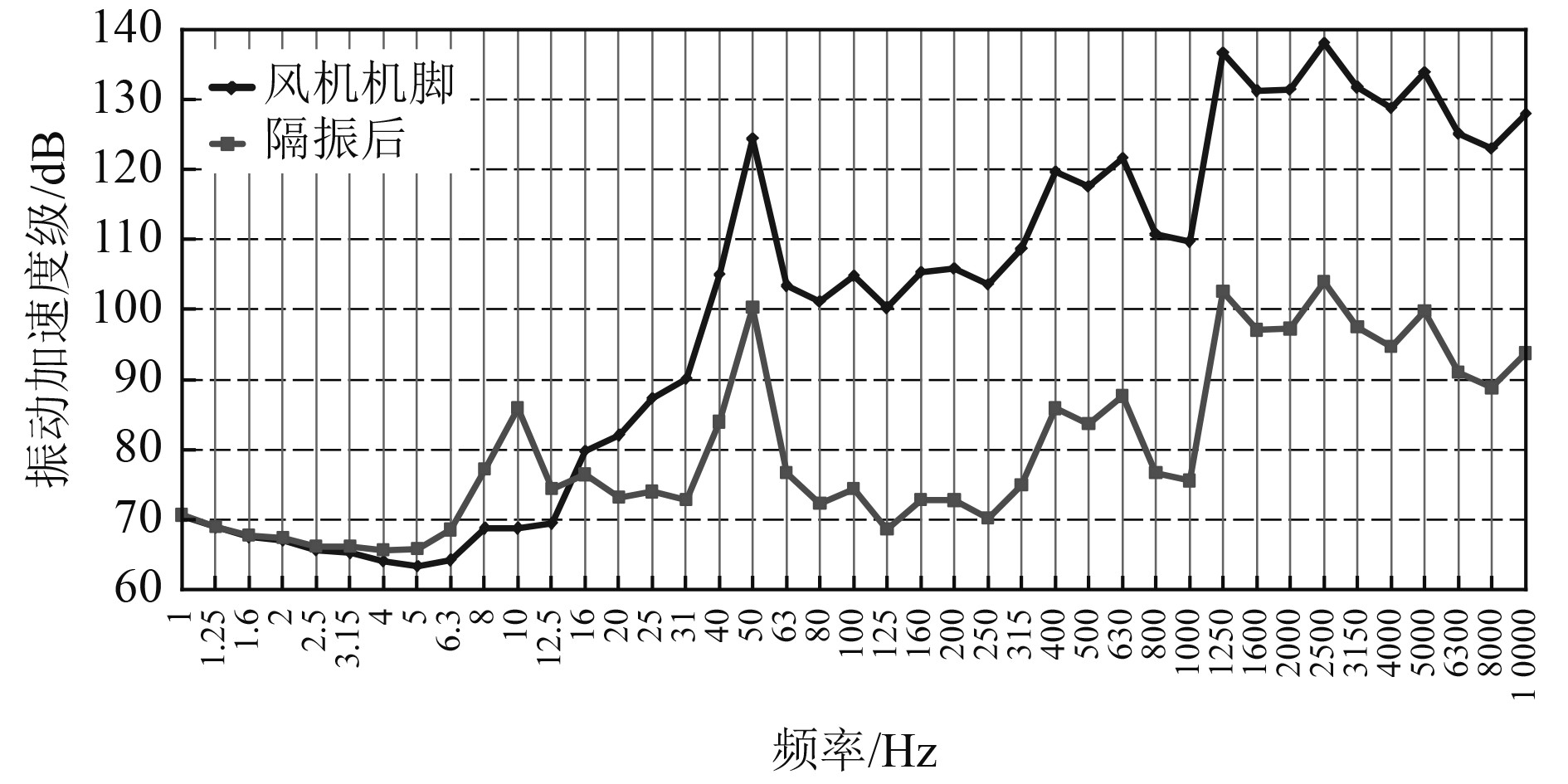图 5 离心风机机脚及隔振后振动加速度级曲线 Fig. 5 Curves of vibration acceleration level of feet and foundation

3 空调器其他振动控制研究

3.1 空调器整体单层隔振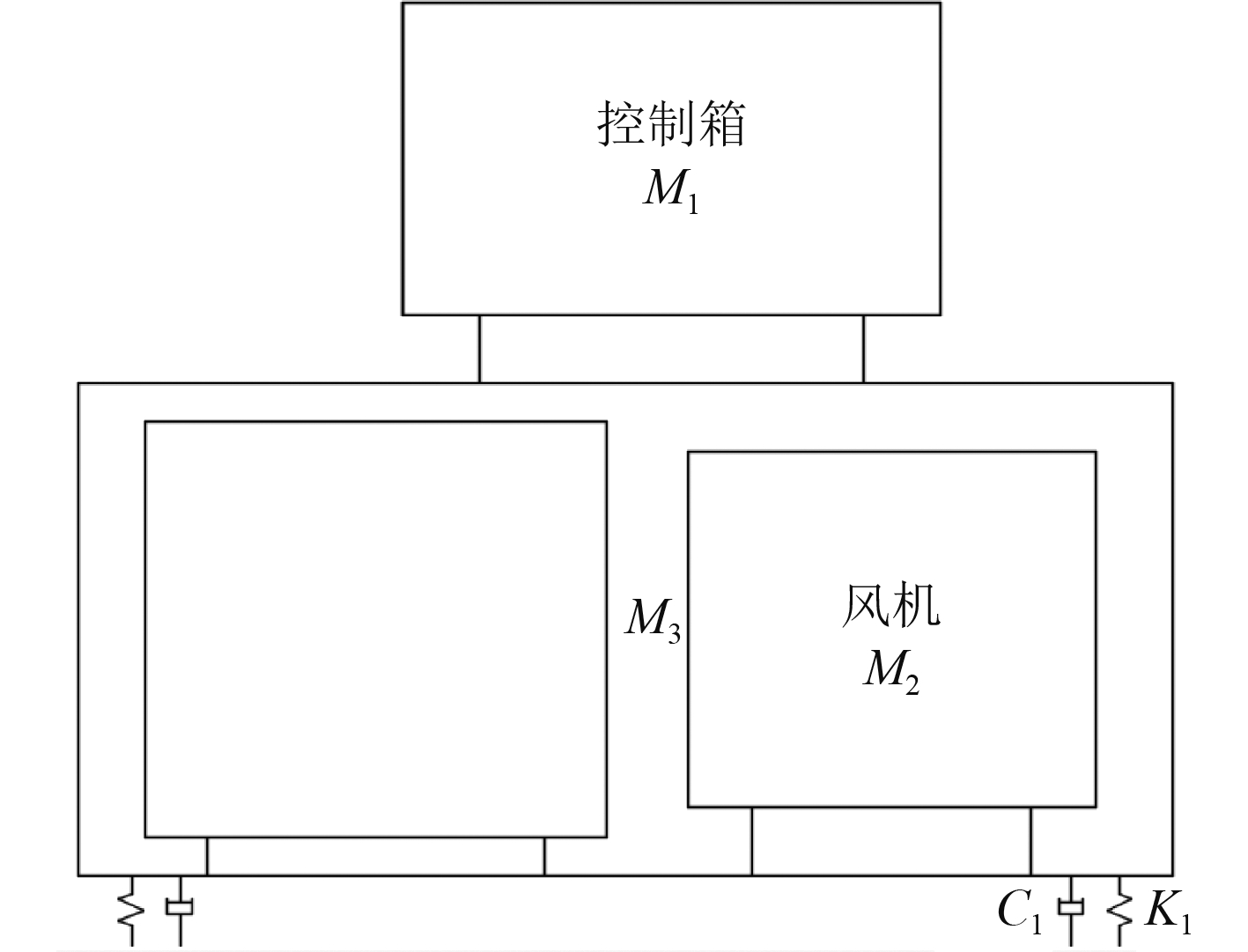图 6 空调器整体单层隔振模型 Fig. 6 Model of signal layer vibration isolation of air conditioner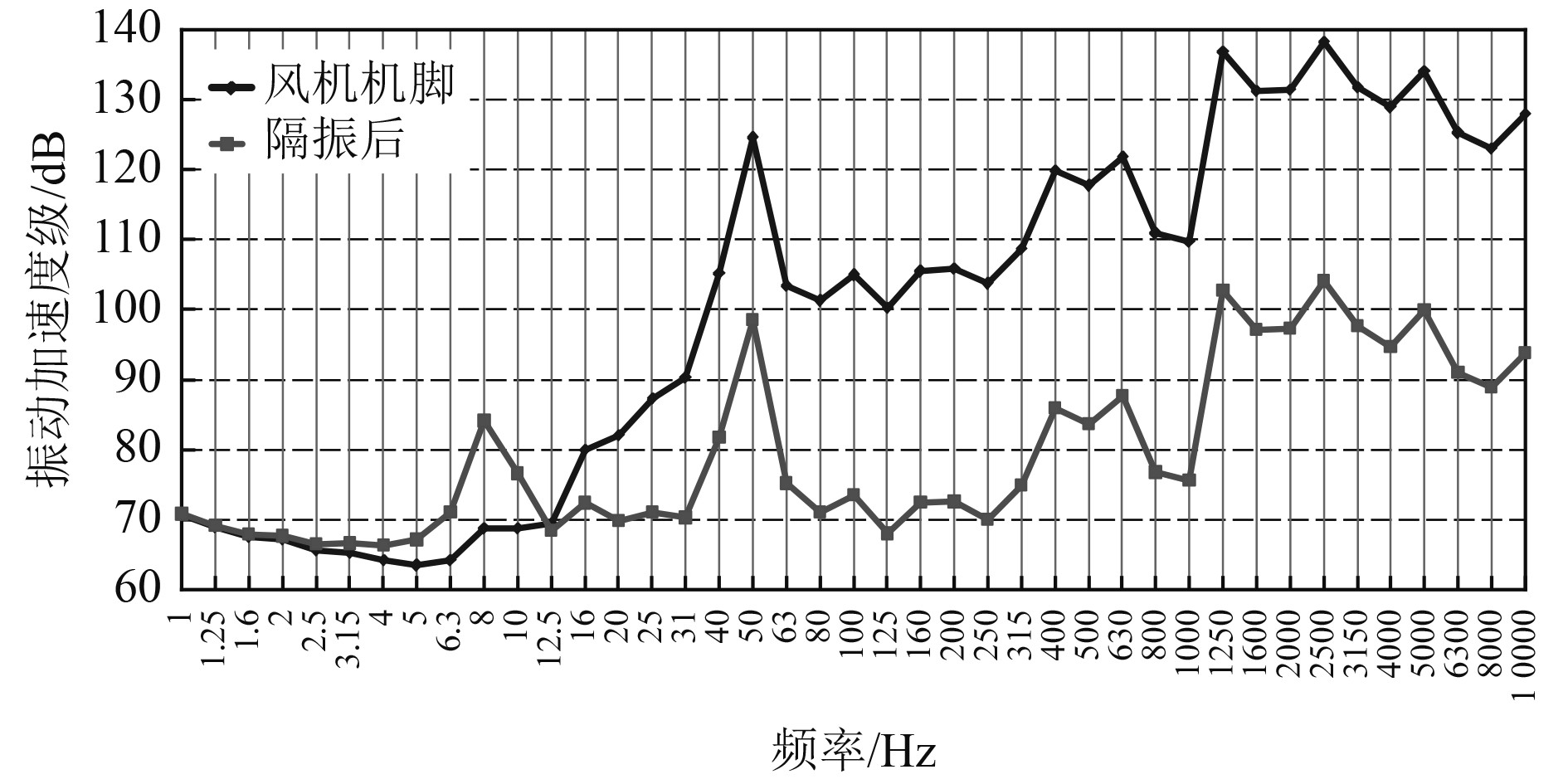图 7 离心风机机脚及空调器隔振后振动加速度级曲线 Fig. 7 Curves of vibration acceleration level of feet and foundation
3.2 空调器双层隔振系统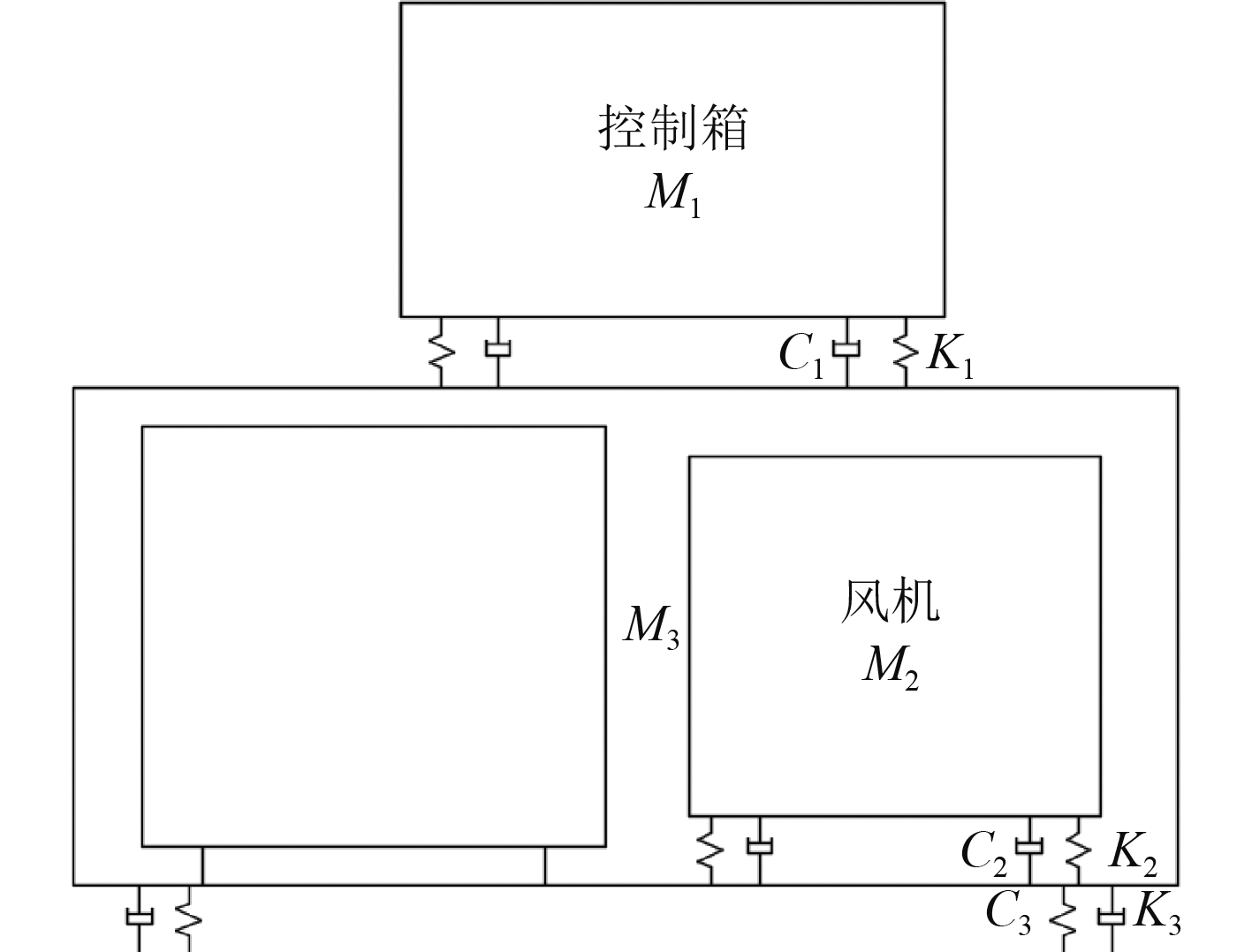图 8 空调器双层隔振模型 Fig. 8 Model of double layer vibration isolation of air conditioner

 $\begin{array}{l} {{{M}}_2}{{\ddot x}_2} + {{{K}}_2}\left( {{{{x}}_{2.}}{{ - }}{{{x}}_3}} \right){{ = }}{{{F}}_0}\sin \omega {{t}}\text{，}\\ {{{M}}_3}{{\ddot x}_3} + {{{K}}_2}\left( {{{{x}}_3}{{ - }}{{{x}}_2}} \right) + {{{K}}_3}{{{x}}_3}{{ = }}0\text{。} \end{array}$ (3)

 $T = \frac{{{{{F}}_{T{{0}}}}}}{{{{{F}}_0}}} = \left| {\frac{1}{{\displaystyle\frac{1}{{\omega _2^2\omega _3^2}}{\omega ^4} - \left( {\displaystyle\frac{1}{{\omega _2^2}} + \displaystyle\frac{1}{{\omega _3^2}} + \mu \displaystyle\frac{1}{{\omega _3^2}}} \right){\omega ^2} + 1}}} \right|\text{。}$ (4)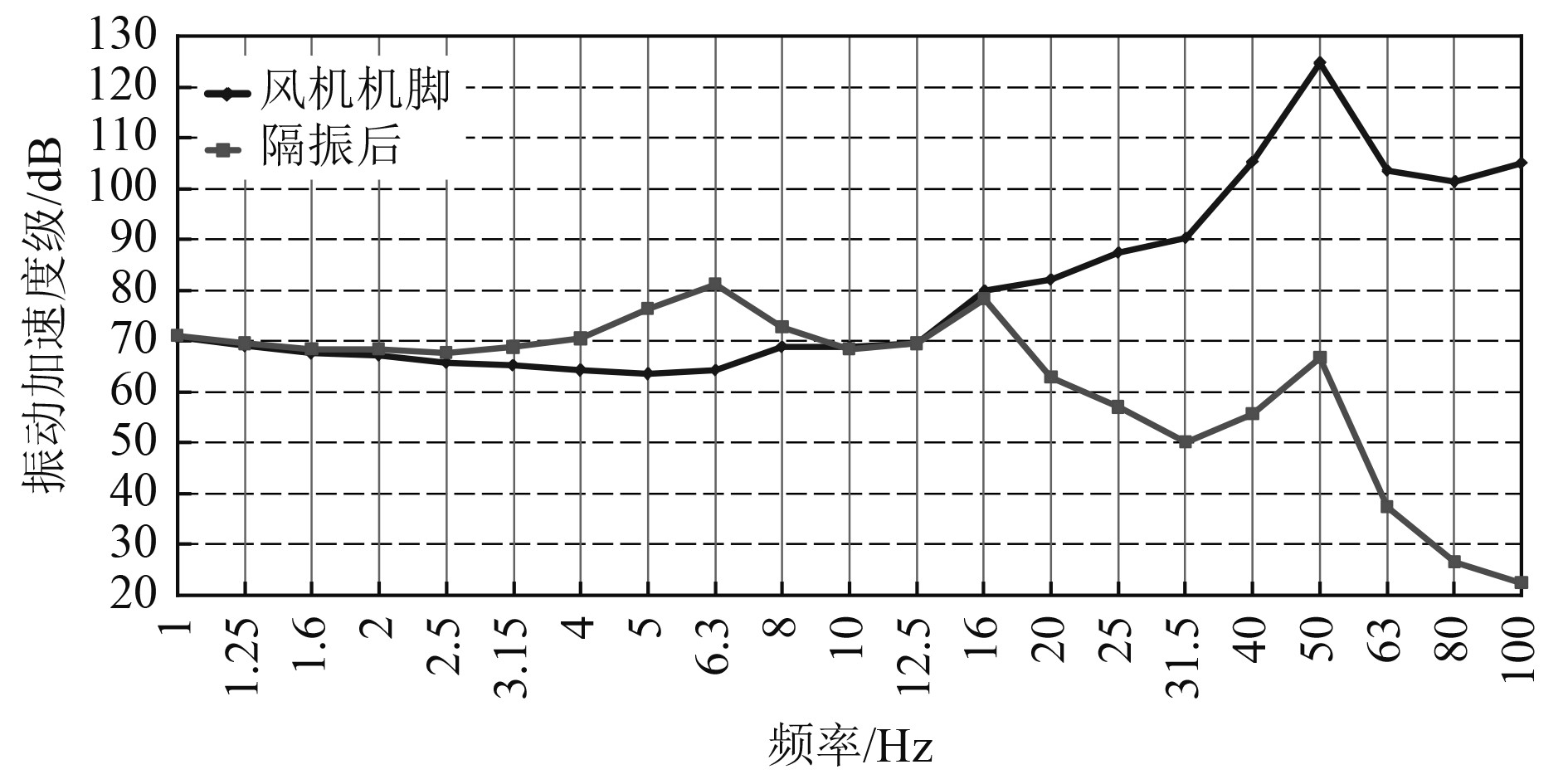图 9 离心风机机脚及空调器双层隔振后振动加速度级曲线 Fig. 9 Curves of vibration acceleration level of feet and foundation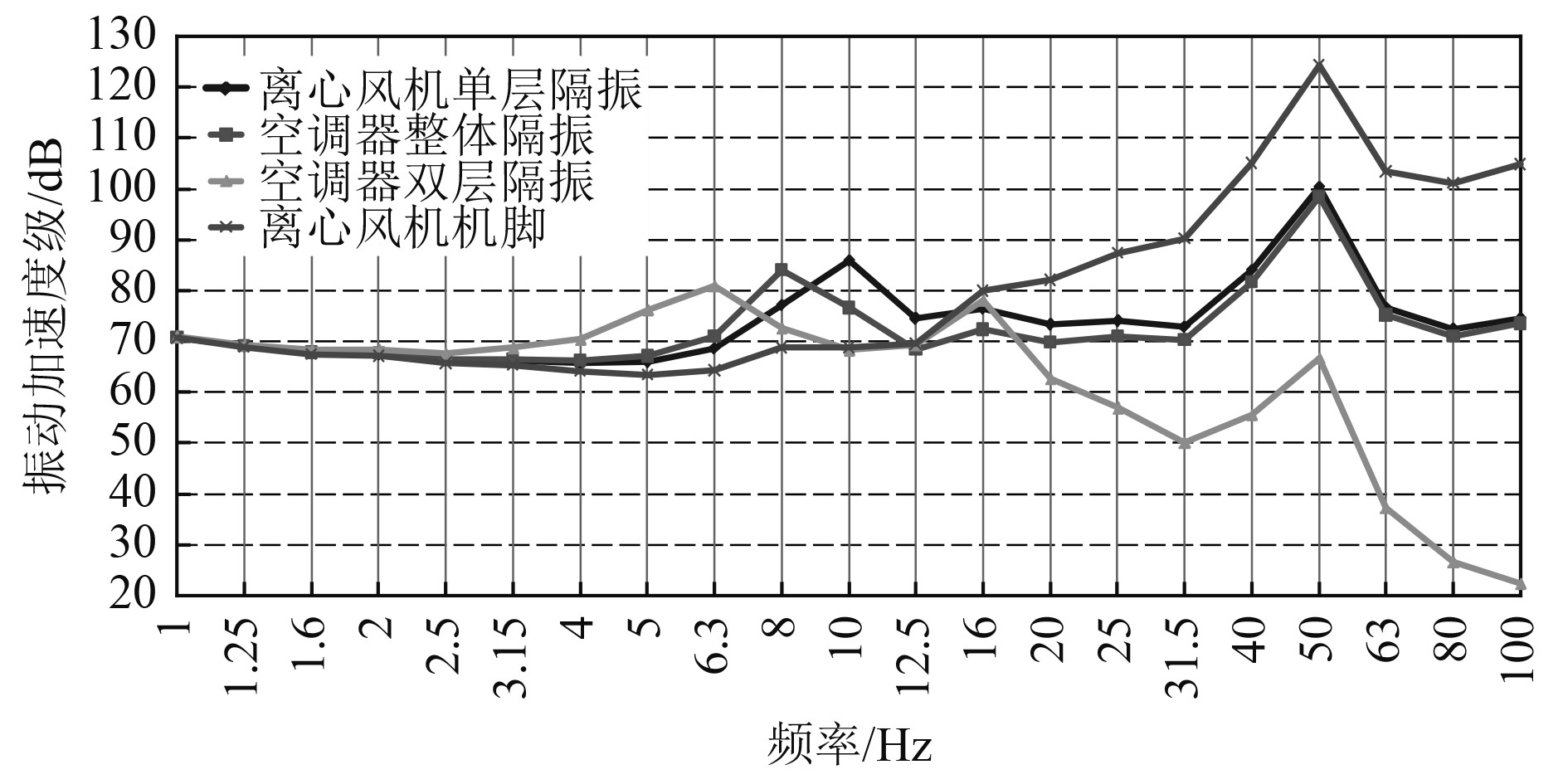图 10 不同方案1～100 Hz振动加速度级曲线 Fig. 10 Curves of vibration acceleration level of foundation with different conditions
4 结　语

1）空调器采取离心风机隔振，空调器整体隔振及空调器双层隔振等措施，均可有效降低振动传递至安装基础，其中空调器双层隔振等的隔振效率最佳。

2）在现有方案基础上，对空调器整体采取隔振措施形成双层隔振系统，将有利于隔离空调器的低频振动传递至安装基础，有利于舰船的低频振动控制。

  董国良. 变频组合式空调装置在船舶上的应用及发展前景[J]. 机电设备, 2006(02): 1-4+8.  王广夫, 张磊, 戴君煜, 等. 船舶空调器室内离心风机的降噪技术研究[J]. 机电设备, 2017, 34(01): 13-18.  由成良. 辅助船空调、通风系统的噪声分析与控制[J]. 船舶, 2012, 23(06): 48-53. DOI:10.3969/j.issn.1001-9855.2012.06.009  武耀, 季振林, 王笃勇. 船用柜式空调器噪声源识别与分析[J]. 声学与电子工程, 2011(04): 40-42+53.  管卫华, 林用满, 卢锦程, 等. 船舶舱室空调通风管路减振降噪方法研究[J]. 舰船科学技术, 2018, 40(14): 10-12.  董鹏, 李志印, 简弃非. 大温差变风量送风技术在潜艇空调中的应用[J]. 中国舰船研究, 2012, 7(06): 90-97.